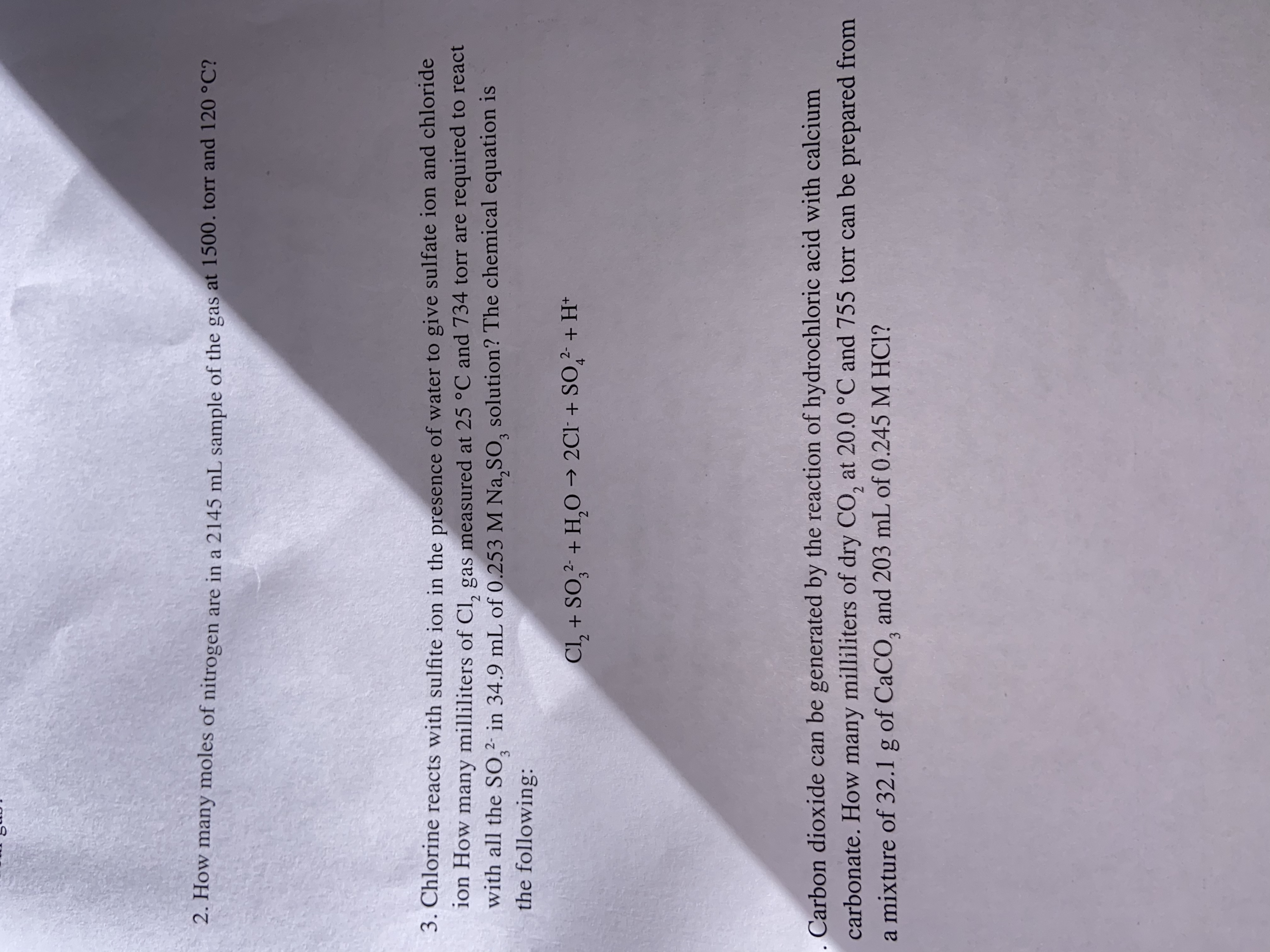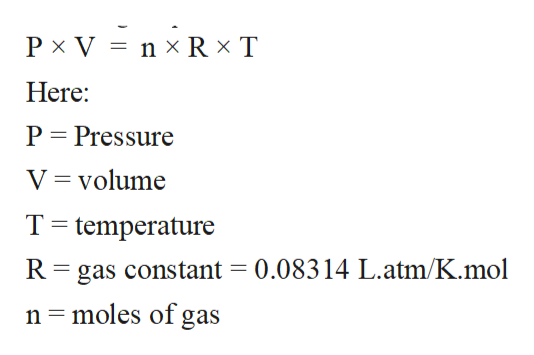# 2. How many moles of nitrogen are in a 2145 mL sample of the gas at 1500. torr and 120 °C?3. Chlorine reacts with sulfite ion in the presence of water to give sulfate ion and chlorideion How many milliliters of CI, gas measured at 25 °C and 734 torr are required to reactwith all the S0,2 in 34.9 mL of 0.253 M Na, SO, solution? The chemical equation isthe following:Cl2+ SO2+ H,O 2Cl +SO2+H*43Carbon dioxide can be generated by the reaction of hydrochloric acid with calciumcarbonate. How many milliliters of dry CO, at 20.0 °C and 755 torr can be prepared froma mixture of 32.1 g of CaCO, and 203 mL of 0.245 M HCI?

Question

I need help with these three questions, they are all interconnected so I grouped them as one.help_outlineImage Transcriptionclose2. How many moles of nitrogen are in a 2145 mL sample of the gas at 1500. torr and 120 °C? 3. Chlorine reacts with sulfite ion in the presence of water to give sulfate ion and chloride ion How many milliliters of CI, gas measured at 25 °C and 734 torr are required to react with all the S0,2 in 34.9 mL of 0.253 M Na, SO, solution? The chemical equation is the following: Cl2+ SO2+ H,O 2Cl +SO2+H* 4 3 Carbon dioxide can be generated by the reaction of hydrochloric acid with calcium carbonate. How many milliliters of dry CO, at 20.0 °C and 755 torr can be prepared from a mixture of 32.1 g of CaCO, and 203 mL of 0.245 M HCI? fullscreen
check_circleExpert Solution
Step 1

We’ll answer the first question since the exact one wasn’t specified. Please submit a new question specifying the one you’d like answered.

All matter can be classified as solid, liquid and gases mainly. In these physical states of matter, the solid state is composed of particles with least intermolecular distance and strongest intermolecular force of attraction. On the contrary, the gaseous state of matter is composed of particles with largest intermolecular distance and weakest intermolecular force of attraction. The liquid state of matter exhibits the intermediate properties of them.

Step 2

The gaseous state is the state of matter with least intermolecular force of attraction between molecules and highest kinetic energy. The standard properties of gases like Pressure, volume, and temperature can be calculated with the help of ideal gas equation.

Step 3

The ideal gas equation c...help_outlineImage TranscriptionclosePx V n x Rx T Here: P Pressure V volume T temperature R gas constant = 0.08314 L.atm/K.mol n moles of gas fullscreen

### Want to see the full answer?

See Solution

#### Want to see this answer and more?

Solutions are written by subject experts who are available 24/7. Questions are typically answered within 1 hour*

See Solution
*Response times may vary by subject and question
Tagged in

### Ideal gases